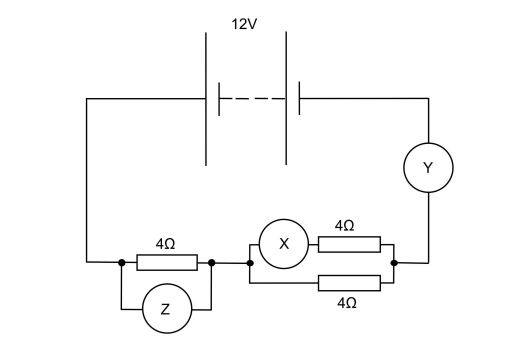# IMAT 2014 Q60 [Circuits]

The circuit shown contains three identical resistors, two ammeters X and Y, and a voltmeter Z.

The internal resistance of the battery is negligible.Which option shows the readings on the three meters?

[Assume the ammeters have negligible resistance, and negligible current flows through the voltmeter.]

A. X = 1.0 A; Y = 0.0 A; Z = 12 V
B. X = 2.0 A; Y = 0.0 A; Z = 4.0 V
C. X = 1.0 A; Y = 2.0 A; Z = 8.0 V
D. X = 3.0 A; Y = 6.0 A; Z = 12 V
E. X = 1.0 A; Y = 2.0 A; Z = 6.0 V

In order to solve this question, we need to figure out what is the equivalent resistor: R_e.

Certain rules need to be remembered:

a) n resistors connected in series: R_e = R_1+R_2+\dotsc + R_n

b)m resistors connected in parallel: \frac{1}{R_e} =\frac{1}{R_1}+\frac{1}{R_2} + \dotsc +\frac{1}{R_m}

tip:
In the case when the values of the resistors are the same, it is nice to know that for 2 resistors with the value R in series, the equivalent is 2 \cdot R the resistor value, but when the resistors are in parallel, the equivalent is \frac{1}{2} \cdot R .

So in this situation, the equivalent resistance is R_e = 4\Omega + \frac{1}{2} \cdot 4\Omega = 4\Omega + 2\Omega = 6\Omega

In order to get the current value in Y we need to take the voltage and divide it by the equivalent resistance: I_Y = \frac{12V}{R_e} =\frac{12V}{6\Omega}=2A

Having this in mind, we will automatically cross the solutions (A), (B) and (D) and incorrect.

Looking at the solutions (B) and (E) we can see that the difference is in the Z meter, so we will calculate this value.

To calculate the voltage on Z we need to know the rules of the voltage divider:

V_Z = \frac{R_Z}{R_e} \cdot 12V = \frac{4\Omega}{6\Omega} \cdot 12V = 4 \cdot 2V = 8V \to V_Z = 8V

Which means that the right solution is (C)

Resistive voltage divider:

V_{out} = \frac{R_2}{R_1 + R_2} \cdot V_{in}